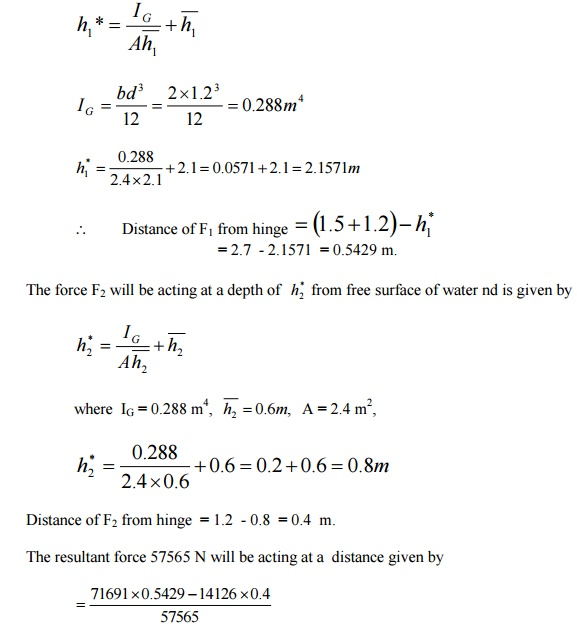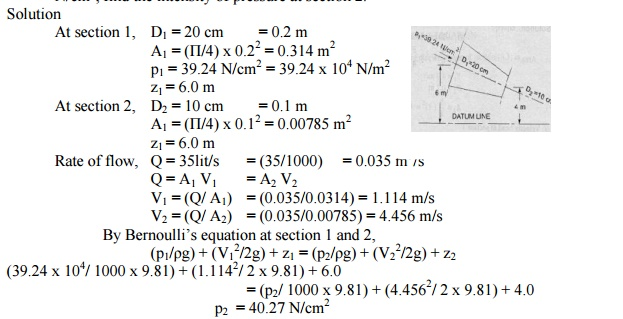Home | | Mechanics of Fluids | Solved Problems: Civil - Fluid Kinematics And Dynamics

# Solved Problems: Civil - Fluid Kinematics And Dynamics

Civil - Mechanics Of Fluids - Fluid Kinematics And Dynamics

Problem 1

A U-Tube manometer is used to measure the pressure of water in a pipe line, which is in excess of atmospheric pressure. The right limb of the manometer contains water and mercury is in the left limb. Determine the pressure of water in the main line, if the difference in level of mercury in the limbs U. U tube is 10 cm and the free surface of mercury is in level with over the centre of the pipe. If the pressure of water in pipe line is reduced to 9810 N/m2, Calculate the new difference in the level of mercury. Sketch the arrangement in both cases.

Given,

Difference of mercury  = 10 cm = 0.1 m.

Let PA = pr of water in pipe line (ie, at point A)

The point B and C lie on the same horizontal line. Hence pressure at B should be equal to pressure at C.

But pressure at B = Pressure at A and Pressure due to 10 cm (or) 0.1m of water.

=PA +p g h

where , P = 1000kg/m3 and h = 0.1 m

=PA  +1000 9.810.1

=PA  +981N / m 2Problem 2:

A differential manometer is connected at the two points A and B of two pipes a shown in figure. The pipe A contains a liquid of sp. Gr = 1.5 while pipe b contains a liquid of sp . gr = 0.9 .  The pressures at A and B are 1 kgf / cm2 respectively. Find  the  difference  in mercury level  in  the  differential manometer.Problem 3

A vertical sluice gate is used to cover an opening in a dam. The opening is 2m wide and 1.2m high. On the upstream of the gate, the liquid of sp. Gr

1.45, lies upto a height of 1.5m above the top of the gate, whereas on the downstream side the water is available upto a height touching the top of the gate. Find the resultant force acting on the gate and position of centre of pressure. Find also the force acting horizontally at the top of the gate and posiotn of centre of pressure. Find also the force acting horizontally at the

top of the gate which is capable of opening it. Assume the gate is hinged at the bottom.Problem 4

Find the density of a metallic body which floats at the interface of mercury of sp. Gr 13.6 and water such that 40% of its volume is sub-merged in

mercury and 60% in water.5.     A solid cylinder of diameter 4.0 m has a height of 3m. Find the meta - centric height of the cylinder when it is floating in water with its axis vertical. The sp gr of the cylinder -0.6 .Problem 6

A wooden cylinder of sp. Gr = 0.6 and circular in cross -section is required to float in oil (sp.gr = 0.90) . Find the L/D ratio for the cylinder to float with its longitudinal axis vertical in oil, where L is the height of cylinder and D is

its diameters.

Solution:

Dia of cylinder  =  D

Height of cylinder  = L

Sp gr of cylinder, S1 = 0.6

Sp gr of oil, S2 = 0.9

Let, depth of cylinder immersed in oil = h

Buoyancy principle

Weight of cylinder = Weight of oil dispersed.Problem 7

In  a  two  -two  dimensional  incompressible  flow, the fluid velocity

components are given by u = x -4y and v= - y -4x.

show that velocity potential exists and determine its form. Find also the stream function.SECTION 2

1.A pipe through which water is flowing is having diameters 20 cm and 10 cm at the cross-sections 1 and 2 respectively. The velocity of water at section 1 is given 4 m/s. Find the velocity head at sections 1 and 2 and also rate of discharge.2.The water is flowing through a pipe having diameter 20 cm and 10 cm at sections 1 and 2 respectively. The rate of flow through pipe is 35 litres/s. The section 1 is 6 m above datum and section 2 is 4 m above datum. If the pressure at section 1 is 39.24 N/cm2, find the intensity of pressure at section 2.3.Water is flowing through a pipe having diameter 300 mm and 200 mm at the bottom and upper end respectively. The intensity of pressure at the bottom end is 24.525 N/cm2 and the pressure at the upper end is 9.81 N/cm2. Determine the difference in datum head if the rate of flow through pipe is 40 lit/s.4.In a two dimensional incompressible flow the fluid velocity components are given by u = x - 4y and v = -y - 4x, Where u and v are x and y components of flow velocity. Show that the flow satisfies the continuity equation and obtain the expression for stream function. If the flow is potential, obtain also the expression for the velocity potential.5.The stream function and velocity potential for a flow are given by ? = 2xy and ? = x2 - y2. Show that the conditions for continuity and irrotational flow are satisfied. Solution:From the properties of Stream function, the existence  of stream function shows the possible  case   of  flow       and  if  it  satisfies  Laplace    equation,  then the  flow  is irrotational.

5. A 250 mm diameter pipe carries oil of specific gravity 0.9 at a velocity of 3 m/s. At another section the diameter is 200 mm. Find the velocity at this section and the mass rate of flow of oil.9.A 30 cm x 15 cm venturimeter is inserted in a vertical pipe carring water, flowing in a upward direction. A differential mercury manometer connected to the inlet and throat gives a reading of 20 cm. Find the discharge. Take Cd = 0.98.Study Material, Lecturing Notes, Assignment, Reference, Wiki description explanation, brief detail
Civil : Mechanics Of Fluids : Fluid Kinematics And Dynamics : Solved Problems: Civil - Fluid Kinematics And Dynamics |